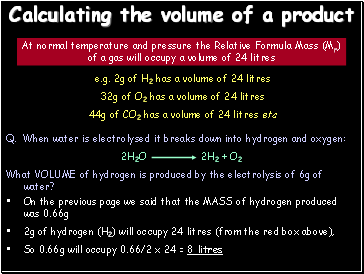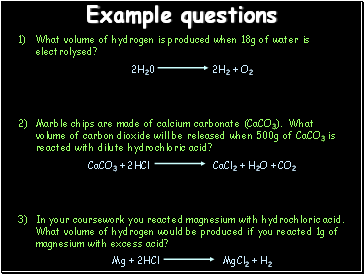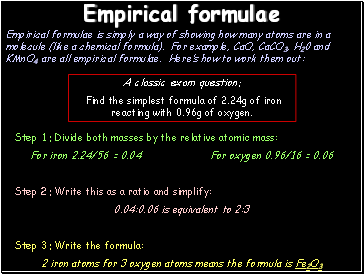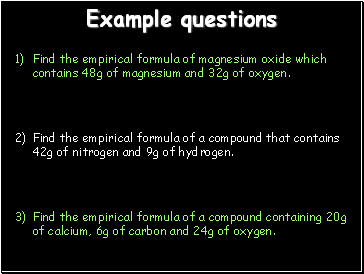# Mass, atomic and empirical formulasPage 2

#### WATCH ALL SLIDES

Try using this equation:

Mass of product IN GRAMMES

6g

4

36

So mass of product = (4/36) x 6g = 0.66g of hydrogen

Slide 9## Calculating the volume of a product

At normal temperature and pressure the Relative Formula Mass (Mr) of a gas will occupy a volume of 24 litres

e.g. 2g of H2 has a volume of 24 litres

32g of O2 has a volume of 24 litres

44g of CO2 has a volume of 24 litres etc

On the previous page we said that the MASS of hydrogen produced was 0.66g

2g of hydrogen (H2) will occupy 24 litres (from the red box above),

So 0.66g will occupy 0.66/2 x 24 = 8 litres

Slide 10## Example questions

What volume of hydrogen is produced when 18g of water is electrolysed?

2H20 2H2 + O2

Marble chips are made of calcium carbonate (CaCO3). What volume of carbon dioxide will be released when 500g of CaCO3 is reacted with dilute hydrochloric acid?

CaCO3 + 2HCl CaCl2 + H2O + CO2

In your coursework you reacted magnesium with hydrochloric acid. What volume of hydrogen would be produced if you reacted 1g of magnesium with excess acid?

Mg + 2HCl MgCl2 + H2

Slide 11## Empirical formulae

Empirical formulae is simply a way of showing how many atoms are in a molecule (like a chemical formula). For example, CaO, CaCO3, H20 and KMnO4 are all empirical formulae. Here’s how to work them out:

A classic exam question:

Find the simplest formula of 2.24g of iron reacting with 0.96g of oxygen.

Step 1: Divide both masses by the relative atomic mass:

For iron 2.24/56 = 0.04 For oxygen 0.96/16 = 0.06

Step 2: Write this as a ratio and simplify:

0.04:0.06 is equivalent to 2:3

Step 3: Write the formula:

2 iron atoms for 3 oxygen atoms means the formula is Fe2O3

Slide 12## Example questions

Find the empirical formula of magnesium oxide which contains 48g of magnesium and 32g of oxygen.

Find the empirical formula of a compound that contains 42g of nitrogen and 9g of hydrogen.

Find the empirical formula of a compound containing 20g of calcium, 6g of carbon and 24g of oxygen.

Go to page:
1  2